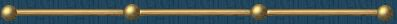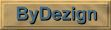MixProps:  Pump Example

The feed to a pump has the following composition:

 Component Composition (mass %) Hydrogen 0.0200 Methane 1.1418 Benzene 71.7223 Toluene 27.1159 Total: 100.0000

(NoteEngVert can easily convert mol/mass percentages and fractions of streams.)

The feed to the pump is at T = 70 F and reservoir P = 485 psia.  Assume the following layout of the pump:

Liquid height above pump eye, z = 3 feet
Suction line head loss, h =  3.5 feet

The Operations Manager swears that the pump will cavitate due to the light ends present (hydrogen and methane), low liquid head height, and suction line losses...  Is he right?

Compute the NPSHa (net positive suction head, available) to the pump.  The NPSHa is defined as:

NPSHa = Pr -Pvp + z - h

where:

Pr = reservoir pressure = 485 psia
Pvp = vapor pressure = ???
z = liquid height above pump eye = 3 feet
h = suction line head loss = 3.5 feet

NOTE:  To ease data entry, this flash calculation file is stored in the directory where MixProps is installed...

Step 1:  On the Mixprops "Settings" tab, perform a bubble-point pressure calculation at T = 70 F.  Select the SRK-78 (API) EOS model.

Step 2:  On the Mixprops "Composition" tab, enter the above pump suction composition.  NOTE:  Since the pump feed contains hydrogen and the EOS being used is the SRK-78 (API), use the MixProps special component HYDROGEN (API).

Step 3:  Click on the "Interaction Coefficients" tab.  Since the pump suction conditions are at a relatively high pressure, click on the "Estimate Interactions Coefficients" check-box.  MixProps will automatically estimate the binary interactions for the components.

Step 4:  Click on the MixProps "Properties" tab.  Mixprops performs the BUBP calculation (note the liquid phase fraction = 1.0000) and computes all the thermodynamic and transport properties of the pump feed.  If the suction line head loss was not given, an application such as PipeDrop could be used to compute the line loss.

Step 5:  Click on the MixProps "Summary" tab.  Read off the bubble pressure = "vapor pressure" =  445.18 psia.  Note that the liquid density = 52.2 lbm/ft3 and the specific gravity relative to water = 52.2 / 62.4 = 0.8365

Step 6:  Compute the NPSHa to the pump:

NPSHa = [(485 - 445.18)2.31] / 0.8365 + 3 - 3.5 = 109.5 feet (sufficient to avoid cavitation for any pump)

Gently inform the Operations Manager that he has nothing to worry about...Graphics by:©1999-2005 by G&P Engineering Software Last Modified:  Sept 30, 2005